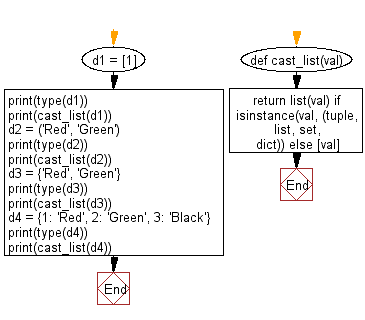﻿ Python: Casts the provided value as a list if it's not one - w3resource# Python: Casts the provided value as a list if it's not one

## Python List: Exercise - 266 with Solution

Write a Python program to cast the provided value as a list if it's not one.

• Use isinstance() to check if the given value is enumerable.
• Return it by using list() or encapsulated in a list accordingly.

Sample Solution:

Python Code:

``````def cast_list(val):
return list(val) if isinstance(val, (tuple, list, set, dict)) else [val]
d1 = 
print(type(d1))
print(cast_list(d1))
d2 = ('Red', 'Green')
print(type(d2))
print(cast_list(d2))
d3 = {'Red', 'Green'}
print(type(d3))
print(cast_list(d3))
d4 = {1: 'Red', 2: 'Green', 3: 'Black'}
print(type(d4))
print(cast_list(d4))
```
```

Sample Output:

```<class 'list'>

<class 'tuple'>
['Red', 'Green']
<class 'set'>
['Green', 'Red']
<class 'dict'>
[1, 2, 3]
```

Flowchart:## Visualize Python code execution:

The following tool visualize what the computer is doing step-by-step as it executes the said program:

Python Code Editor:

Have another way to solve this solution? Contribute your code (and comments) through Disqus.

What is the difficulty level of this exercise?

Test your Python skills with w3resource's quiz

﻿

## Python: Tips of the Day

Floor Division:

When we speak of division we normally mean (/) float division operator, this will give a precise result in float format with decimals.

For a rounded integer result there is (//) floor division operator in Python. Floor division will only give integer results that are round numbers.

```print(1000 // 300)
print(1000 / 300)```

Output:

```3
3.3333333333333335```